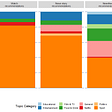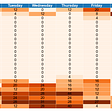# Understanding Bayesian linear regression & Gaussian process with Normal Distributions

In the last article, we learn the normal distribution properties that are central to Machine Learning (ML). Here, we will apply our knowledge in manipulating normal distributions to solve the intractable Bayesian inference. And we will demonstrate it with the Bayesian linear regression and the Gaussian process. We will also go through a few proofs to apply what we learn. But we assume you have read the last article, please do so if you have not done it.

# Bayesian linear regression

Bayesian linear regression takes advantage of the “convenience” of normal distribution operations and solves the regression problem analytically.

For a dataset with a sample size of n and each data point has m features, Bayesian linear regression is defined as

And both the prior over parameters θ and the error ε are assumed to be normally distributed.

Posterior

When manipulating normal distributions, if the result is a probability distribution, we can focus on merging these normal distributions and ignore any scaling factors. We can focus on finding the parameters of the resulting normal distribution. Here, the posterior equals

i.e. the posterior is

Posterior predictive distribution

To compute the posterior predictive distribution in Bayesian inference, we integrate the posterior over all the possible values θ for the model.

This integration is intractable in general. In the Bayesian linear regression, the likelihood is in the form of a Gaussian function.

Therefore, we can compute the posterior predictive distribution much easier by taking advantage of the normal distribution properties. Recall the Bayesian regression model is defined as

And the linear transformation rule on the normal distribution is.

Let’s replace A with x* and x with θ, we get

Apply the summation rule to account for ε in the Bayesian regression equation

The posterior predictive distribution becomes

Putting everything together, the posterior predictive distribution is

The mean of this distribution is the point estimation for y* given x*.

Next, we will discuss the Gaussian Process that explores relationships between data points with normal distributions.

# Gaussian Process (GP)

Let’s have a quick overview of what GP can do. The distribution of a Gaussian process (GP) is a distribution over functions. What does it mean? Given the training dataset D = {(x₁, y₁), (x₂, y₂), (x₃, y₃), (x₄, y₄)}, function f below fits D exactly.

But a Bayesian never settles down on a point estimate! Indeed, there are an infinite number of functions that can fit these data points exactly. But some functions are more likely than others. For example, function f ² below seems more likely than others as it makes less curvy changes to fit the data. For frequentists, they will use the most likelihood estimation (MLE) to give us a final regression model. But for Bayesians, they model all the possibilities. For demonstration, we can sample from a GP repeatedly in plotting out the functions that Bayesians are predicting.

GP is a generative model. It generates functions (samples) that fit

• the observations, and
• our belief on how data are related and what is their expected value (mean).

In a GP regression, it predicts a normal distribution for y* given x*, i.e. the probability density function for f(x*).

Lazy accountant

Let’s go through an illustration quickly. At the beginning of each quarter, an accountant reports the balance between the account receivable (AR) and the account payable (AP) to the CEO (balance = AR minus AP). At any point in time, AR may be higher than AP or vice versa. Unfortunately, this company only manages to break even, i.e. on average, the balance is zero.

The accountant retired and a smart but lazy accountant, Paul, is hired. Instead of doing his job, he creates a multivariate normal distribution below and generates the balance automatically for the next 20 months.

This distribution makes a lot of sense because the expected value will be 0. Here is a plot on a single sample with the next 20-month balances.

The covariance matrix Σ controls how data points are correlated. Σᵢⱼ equals 0 when data point i is not correlated with data point j. If it is greater than zero, they are. Paul’s choice of Σ is an exponential function on the distance between data points. So balances among neighboring months are similar. s is a scaling factor to replicate the range of the balance. l is a tunable hyperparameter (a.k.a. the kernel width). With a larger l, data points influence neighbors further away. From another perspective, each data point will be influenced by more neighbors. Therefore, the corresponding curve will be smoother.

This scheme is so successful that Paul creates a new model and samples one data point a day for the next 20 months.

The plotted graph is smooth and just looks like a function. So he calls his system a sampler of functions. Every sample is a sample of the function f.

The CFO is impressed with the plot and asks him to redo the monthly balance for the last financial year. He cannot reuse the last function sampler anymore. It will not match the previous quarterly reports.

As discussed before, if we know a multivariate normal distribution, we can re-establish the probability distribution of the missing data given the observed one.

As in this example, the probability distribution for x₁ given x₂=2 is

Paul realizes that too. If he knows f (the last 4 quarter balances), he can recreate the distribution of f* for the last 12 months. The first four entries in his new model are the reported balances at months 1, 4, 7, and 10 (every financial quarter). The next 12 entries (f*) represent the new curve for the last financial year.

The diagram below plots one of the functions sampled.

The original prior defines the mean and the covariance of the functions. It is our prior belief on the expectation value for f and how data are correlated. Given the observations (the four balances), the posterior enforces the constraints that any sampled functions must cross the path at the red dots below.

But sometimes, the CEO is interested in a few data points only, for example, the balance on the 60th and the 280th day. This can be modeled by a new multivariate normal distribution below. It samples a function f* that predicts the outputs for the specific inputs. For instance, one possible sample for f* is [f* ¹(125) = -0.2, f* ¹(300) = 1.1]).

There is another important observation. As we move away from the red dots further, the variance of f increases. The prediction will have a wider range of guesses and lower certainty. In short, as we move away from known data points, the uncertainty of the predictions increases.

GP Definition

A Gaussian process is a normal distribution over functions. Each GP is defined by a mean function m(x) and a covariance function κ(x, x′). κ models the covariance (correlation) between f (x) and f(x’).

Paul designs m(x) to be 0 to reflect the expected balance. If we keep sampling functions, the average on f ¹(x), f ²(x), f ³(x), … approaches m(x).

Paul uses a Gaussian kernel to model the covariance function.

For any pair of x and x’, the sampled data points f(x) and f(x) form a bivariate normal distribution. As a simple demonstration, let’s have x’ equals x. As shown below, κ(x, x), the red curve, is normally distributed.

How do we sample data from a GP? In particular, A function can be viewed as a collection of random variables f(x₁), f(x₂), f(x₃), … For continuous functions, the number of random variables is unlimited. Therefore, it has infinite random variables and GP has an infinite dimension. In practice, we can focus on the k variables in interest, even k can be very large.

Definition: A GP is a collection of random variables in which the joint distribution of every finite subset of random variables is multivariate normally distributed.

By taking advantage of this definition, we model a k-variate normal distribution from the joint distribution. For example, Paul creates the finite subset X = {x₁, x₂, x₃, x₄, …, x₁₂} for the coming year report. It contains 12 random variables holding a balance for each month. Once the 12-variate normal distribution is defined, we sample values from this multivariate normal distribution to create sample functions.

It starts with a prior belief from the designer on how data are related.

Or in Paul’s mind, this is

where

Here are the two possible sample functions returned.

Kernel function

One of the popular kernel functions in modeling the covariance function is the squared exponential kernel

where s is a scaling factor. When xᵢ and xⱼare far apart, k equals 0. If they are identical it equals 1. But there are many choices for the kernel functions. Through experimentation, designers can pick one that models the data the best.

In matrix algebra, matrix K is positive semidefinite if

A covariance matrix is positive semidefinite.

Therefore, a kernel function must be positive semidefinite. It is also symmetrical as Cov(x, x’) = Cov(x’, x).

Posterior predictive distribution

Posterior predictive distribution is about finding f* given x* and D.

The posterior predictive distribution is usually written in the form of

and

By applying the conditional rule on a multivariate normal distribution, the mean vector and the covariance matrix is

This is the noiseless Gaussian process regression.

To account for noise in the information, we formulate the prediction as

With the summation rule on the normal distribution, the noise will be added to the covariance matrix as follows

And the posterior predictive distribution for the normal distribution is

# Next

--

--

--

## More from Jonathan Hui

Deep Learning

Love podcasts or audiobooks? Learn on the go with our new app.

## What you see is what you guess## Python: Detecting Twitter Bots with Graphs and Machine Learning## How can companies get the most out of their data## Data Science Best Practices: Python Environments## Data Visualization in Network Mapping with Kumo.io## Warzone Analysis: Part 5Deep Learning

## Normal Distributions in Machine Learning## The Complexity and Graph Structure of Variable Elimination## Explainable AI: a comprehensive review of the main methods## Why we will never open deep learning’s black box